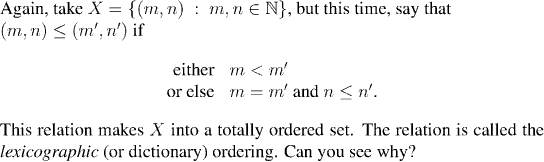Analysis WebNotes
arrow_back arrow_forward

# Class Contents

## Aim: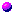Find a set of axioms for the real numbers. These will then be the basis of everything else we do in the course.The axioms will be based on ideas of arithmetic and order.

## Arithmetic

According to our understanding of the reals, we should be able to add, subtract, multiply and divide real numbers.

In other words, the real numbers are a field.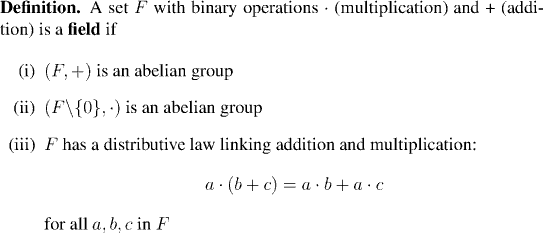One of the axioms of the real numbers will be that they are a field, so let's take a look at some other examples of fields.

Example:

The set Q of rational numbers is a field.

Example:

The set M(2) of 2 by 2 real matrices has addition and multiplication defined on it, but isn't a field. Why not?

Example:

The set {0,1} is a field when addition and multiplication are given by the tables shown:

```. | 0  1          + | 0  1
---------         ---------
0 | 0  0          0 | 0  1
1 | 0  1          1 | 1  0
```

Example:

The set of real numbers (note) :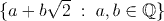is a field.

## Order

The other ingredient we need is order. Given any two real numbers, you can always tell which is bigger. That is to say, the real numbers come in a natural order. There is a relation of "greater than or equal to", and given any pair of real numbers we can test them against this relationship and see whether or not the relationship holds.

Just as the idea of field picks out the important rule for arithmetic, in the same way we now pick out the important rules for the ordering of the real numbers.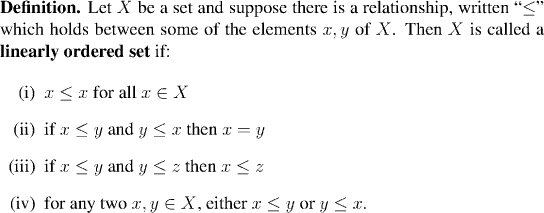Note:

The phrase totally ordered set is sometimes used to mean the same thing as "linearly ordered set".

Example: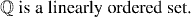Example: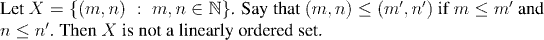Example: